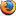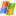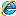### Perl 的四捨五入

```sub round{
my(\$value,\$rank) = @_;
if(\$value>0){
return ( int(\$value * 10**\$rank) + 0.5 ) / 10**\$rank;
}else{
return ( int(\$value * 10**\$rank) - 0.4 ) / 10**\$rank;
}
}
print round(12.345 , 2);```

round() 函式的第一個參數為欲轉換的數值，第二個參數為欲取得的小數點位數

### Javascript 的四捨五入

```<script type='text/javascript'>
//方法一
function round1(value,rank){
if(value >= 0){
return parseInt(value * Math.pow(10,rank) + 0.5) / Math.pow(10,rank);
}else{
return parseInt(value * Math.pow(10,rank) - 0.4) / Math.pow(10,rank);
}
}
//方法二: 使用內建的 round 數學函數
function round2(value,rank){
return Math.round(value * Math.pow(10,rank)) / Math.pow(10,rank);
}
document.write(round1(12.345 , 2) + "<br />");
document.write(round2(12.345 , 2));
</script>```

round1() 及 round2() 函式的第一個參數為欲轉換的數值，第二個參數為欲取得的位數

1.• 晴樹
• 08/21. 2008 9:29上午
UsingMozilla Firefox 3.0 onWindows Vista

很不錯的文章

像有些語言提供現成的round()，其實是『四捨六入五成雙』
判斷方式就有點小差異

2.• cvictor
• 08/26. 2008 12:38上午
UsingMozilla Firefox 2.0.0.16 onWindows XP

負數的處理有點問題喔。還是要減0.5才對。

3.• 小迪
• 10/12. 2011 4:24下午
UsingInternet Explorer 8.0 onWindows XP

呵呵，0.5中间值